C++中真正的临时对象是看不见的，它们不出现在你的源代码中，临时对象的产生在如下几个时刻:

1.       用构造函数作为隐式类型转换函数时，会创建临时对象。

class Integer

{

public:

Integer(int i)

:m_val(i)

{}

~Integer()

{}

private:

int   m_val;

};

void Calculate(Integer itgr)

{

// do something

}

那么语句：  int  i = 10;

Calculate(i);

会产生一个临时对象，作为实参传递到Calculate 函数中。

2.       建立一个没有命名的非堆（non-heap）对象，也就是无名对象时，会产生临时对象。

Integer& iref = Integer(5);           //用无名临时对象初始化一个引用,等价于

//Integer iref(5);

Integer  itgr = Integer(5);           //用一个无名临时对象拷贝构造另一个对象

按理说，C++应先构造一个无名的临时对象，再用它来拷贝构造itgr，由于

Calculate( Integer(5) );                       //无名临时对象作为实参传递给形参,函数调

//用表达式结束后，临时对象生命期结束，被//析构.

3.       函数返回一个对象值时，会产生临时对象，函数中的返回值会以值拷贝的形式拷贝到被调函数栈中的一个临时对象。

Integer Func()

{

Integer itgr;

return itgr;

}

void main()

{

Integer in;

in = Func();

}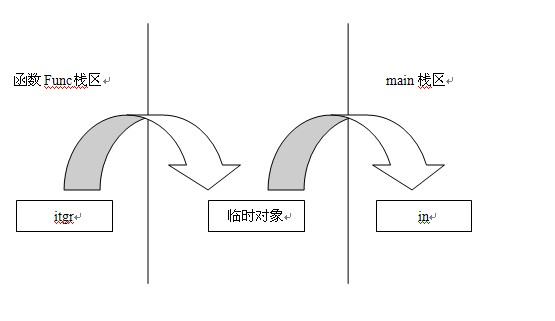Integer& iRef = Func();

class VECTOR3

{

public:

VECTOR3()

:x(0.0f),y(0.0f),z(0.0f)

{

std::cout<<"VECTOR3 Default Constructor "

<<std::setiosflags(std::ios_base::hex)<<this

<<std::endl;

}

VECTOR3(float fx, float fy, float fz)

:x(0.0f),y(0.0f),z(0.0f)

{

std::cout<<"VECTOR3 Parameter Constructor "

<<std::setiosflags(std::ios_base::hex)<<this

<<std::endl;

}

VECTOR3(const VECTOR3& rht)

:x(rht.x), y(rht.y), z(rht.z)

{

std::cout<<"VECTOR3 Copy Constructor "

<<std::setiosflags(std::ios_base::hex)<<this

<<" from rht : "

<<std::setiosflags(std::ios_base::hex)<<&rht

<<std::endl;

}

~VECTOR3()

{

std::cout<<"VECTOR3 Destructor "

<<std::setiosflags(std::ios_base::hex)<<this

<<std::endl;

}

VECTOR3& operator = (const VECTOR3& rht)

{

if( &rht == this )

return *this;

x = rht.x;

y = rht.y;

z = rht.z;

std::cout<<"VECTOR3 operator = left oper : "

<<std::setiosflags(std::ios_base::hex)<<this

<<" right oper : "

<<std::setiosflags(std::ios_base::hex)<<&rht

<<std::endl;

return *this;

}

private:

float x;

float y;

float z;

};

VECTOR3 Func1()

{

return VECTOR3(1.0f, 1.0f, 1.0f);

}

VECTOR3 Func2()

{

VECTOR3 ret;

ret.x = 2.0f;

ret.y = 2.0f;

ret.z = 2.0f;

return ret;

}

void main()

{

VECTOR3 v1 = Func1();

v1 = Func1();

VECTOR3 v2 = Func2();

VECTOR3 v3;

v3 = Func2();

}

<1>.

VECTOR3 v1 = Func1();

1>. Func1() 中构造一个无名对象

2>. Func1() 中的无名对象拷贝构造调用表达式处的临时对象

3>. 再由临时对象拷贝构造v1

4>. Func1() 返回，析构无名对象

5>. 整个语句结束，析构临时对象

1.0f, 1.0f, 1.0f 为参数构造v1，这样只会有一次构造函数的调用。结果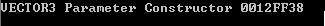<2>.

v1 = Func1();

1>. Func1() 中构造一个无名对象

2>. Func1() 中的无名对象拷贝构造调用表达式处的临时对象

3>. 再由临时对象赋值给v1 (赋值运算符)

4>. Func1() 返回，析构无名对象

5>. 整个语句结束，析构临时对象

1.0f, 1.0f, 1.0f 为参数构造调用表达式处的临时对象，因为是赋值，所以这个临时对象是无法被优化的，赋值完毕后，表达式结束，临时对象被析构。结果如图：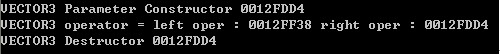<3>.

VECTOR3 v2 = Func2();

1>. Func2() 中的 ret 拷贝构造调用表达式处的临时对象

2>. 该临时对象拷贝构造v2

3>. 析构临时对象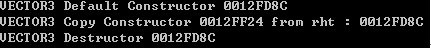<4>.

VECTOR3 v3;

v3 = Func2();

1>. 构造v3

2>. 进入Func2()，构造ret

3>. 返回ret，用ret拷贝构造到调用表达式处的临时对象

4>. Func2()结束，ret被析构

5>. 临时对象赋值给v3

6>. 赋值表达式结束，析构临时对象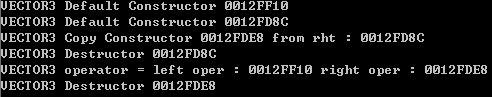<1>. 在使用一个临时对象( 可能是无名对象 或者 返回对象值时 ) 创建构造另一个对象的过程的中，c++会优化掉该临时对象的产生，直接以相同参数调用相关构造函数构或者 直接调用拷贝构造函数 到 目标对象.

<2>. 若不是对象创建，而是对象赋值，则在赋值表达式的右值处的临时对象

创建不能省略，临时对象赋值给左值后，表达式结束，临时对象被析构。

posted on 2010-02-25 14:44 李阳 阅读(3809) 评论(6)  编辑 收藏 引用 所属分类: C++# re: 关于C++中的临时对象问题[未登录] 2011-07-19 01:06 | Leo

# re: 关于C++中的临时对象问题 2013-05-13 16:53 | 教兽

# re: 关于C++中的临时对象问题 2013-07-12 18:02 | PJ

回复  更多评论

# re: 关于C++中的临时对象问题 2013-07-12 18:02 | PJ

# re: 关于C++中的临时对象问题 2013-07-18 15:50 | lite3

# re: 关于C++中的临时对象问题 2014-05-01 03:17 | xmj
Integer &a = Integer( 5 )编译通不过  回复  更多评论